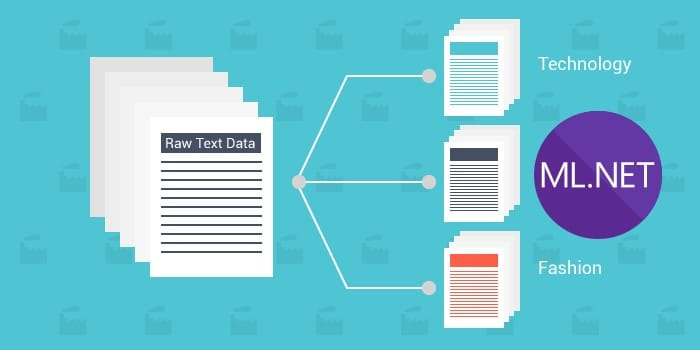# Article Classification with ML.NETToday we are going to learn how to do Article Classification with ML.NET library using the C# language.

You can download the source code at the end of the post.

Since, we’ve already covered Article Classification and K-Means in depth we will focus here mostly on the ML.NET library.

## What is ML.NET?

ML.NET is a machine learning library built for .NET developers by Microsoft. Therefore, It allows you to create custom Machine Learning models without leaving the .NET ecosystem. Best of all, you can re-use all the knowledge you already have as a .NET developer to integrate Machine Learning into your .NET projects.

## ML.NET Workflow

The primary class in ML.NET is a machine learning model. Therefore, we will use this model to read in our data and transform them into a prediction.

In order to do that, we must take the following steps:

• Collect and load our training data into IDataView
• Specify a pipeline of operations to extract features and apply a machine learning algorithm
• Train a model by calling Fit() on the pipeline
• Make predictions by calling CreatePredictionsEngine.Predict()

## Article Classification with ML.NET

This project demonstrates how to use the ML.NET library and it’s features by creating a very simple Article Classification app.

Although, the application is quite simple it shows you how to load your data, create a pipeline of operations, train and create a prediction engine.

## Collect and load our training data into IDataView

For the purposes of this project I collected 18 paragraphs from Wikipedia articles on three topics: Basketball, Movies and Programming Languages.

For example, here are couple of Wikipedia articles:

• Movies
• Programming Languages

Each article is represented by the following class:

``````public class TextData
{
public string Text { get; set; }
}``````

Consequently, all the articles are loaded up into an `IEnumerable<TextData>`

Once, we’ve prepared the data, we can create our machine learning model.

``var mlContext = new MLContext();``

Next, we need to load up the data into a IDataView

``var textDataView = mlContext.Data.LoadFromEnumerable(data);``

## Specify a pipeline of operations to extract features and apply a machine learning algorithm

In order to classify Wikipedia articles we first need to process the text. This is because the K-Means algorithm needs `float[]` type as input. Therefore, we need to transform the text into numerical values.

Let’s see how to do that.

``````var textEstimator = mlContext.Transforms.Text.NormalizeText("Text")
.Append(mlContext.Transforms.Text.TokenizeIntoWords("Text"))
.Append(mlContext.Transforms.Text.RemoveDefaultStopWords("Text"))
.Append(mlContext.Transforms.Conversion.MapValueToKey("Text"))
.Append(mlContext.Transforms.Text.ProduceNgrams("Text"))
.Append(mlContext.Transforms.NormalizeLpNorm("Text"))``````

NormalizeText method allows us to clean up the incoming text by changing the case, removing punctuation marks and numbers.

TokenizeIntoWords method tokenizes the input text (in our case it splits the text into words).

RemoveDefaultStopWords method removes predefined set of text specific to the English language (Stop words in our scenario are: is, this, of, for)

In order to produce a N-Gram we first need to map the words into numerical keys.

MapValueToKey method allows us to dedicate a unique key to each word it appears in the input column.

ProduceNgrams method produces a vector of counts of n-grams (sequences of consecutive words) encountered in the input text

NormalizeLpNorm method scales vectors in the input column to the unit column

As a result, we are ready to attach our K-Means clustering algorithm to the pipeline.

``.Append(mlContext.Clustering.Trainers.KMeans("Text", numberOfClusters: numberOfClusters));``

Where the number of clusters in our scenario would be set to three. Although, in our example it is obvious that we have three categories, sometimes that is not the case. Therefore, you can find the number of clusters by using the Elbow Method.

Let’s look at the complete pipeline code:

``````var textEstimator = mlContext.Transforms.Text.NormalizeText("Text")
.Append(mlContext.Transforms.Text.TokenizeIntoWords("Text"))
.Append(mlContext.Transforms.Text.RemoveDefaultStopWords("Text"))
.Append(mlContext.Transforms.Conversion.MapValueToKey("Text"))
.Append(mlContext.Transforms.Text.ProduceNgrams("Text"))
.Append(mlContext.Transforms.NormalizeLpNorm("Text"))
.Append(mlContext.Clustering.Trainers.KMeans("Text", numberOfClusters:
numberOfClusters));``````

## Train a model by calling Fit() on the pipeline

We can create and train the model by calling Fit() function on the pipeline like so:

``var model = textEstimator.Fit(textDataView);``

where `textEstimator` is out pipeline and `textDataView` is our data representation. Quite simple.

Before we create our prediction engine let’s introduce the `Prediction` class

``````public class Prediction
{
[ColumnName("PredictedLabel")]
public uint Cluster { get; set; }
}``````

This class will hold the prediction result from our prediction engine.

ColumnName attribute allows us to specify IDataView column name directly. This allows us to use the K-Means prediction engine without specifying to which member to assign the prediction result.

Let’s build the prediction engine:

``var predictionEngine = mlContext.Model.CreatePredictionEngine<TextData, Prediction>(model);``

Here we specify the input type and the output type as well as the machine learning model.

As a result, we can now make predictions

``var prediction = predictionEngine.Predict(new TextData() { Text = text });``

## Article Classification with ML.NET application

The demo application is a very simple .NET console app. Once you run it, it will train the model and then allow you to test it.

The first sentence of each category is from our dataset. As a result, we should see the original cluster id assigned to it.

Next, I am typing in a paragraph that is never seen before by our model. And it seems like it is classifying the text correctly.

Please note that K-Means is an unsupervised machine learning algorithm. Therefore, we can not assign labels to our clusters beforehand. The idea behind this algorithm is to use it when you want to find groups of data, which are similar to one another.

And we have just proven that.

Similar data points would stay close to each other. As a result, they create groups, or as we call it clusters.

And indeed, articles/sentences that are similar to each other were assigned the same cluster id.

## Article Classification with ML.NET source code

Article Classification with ML.NET solution